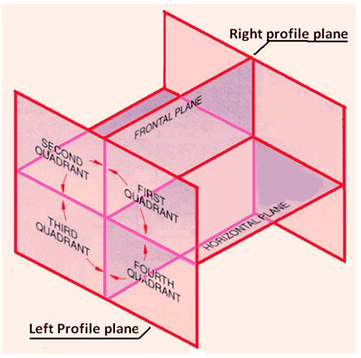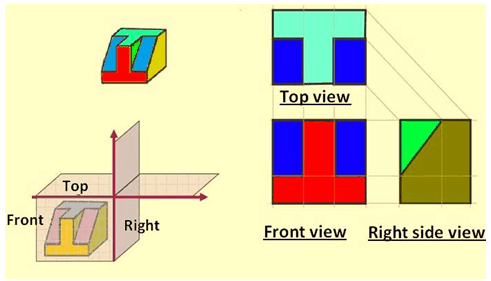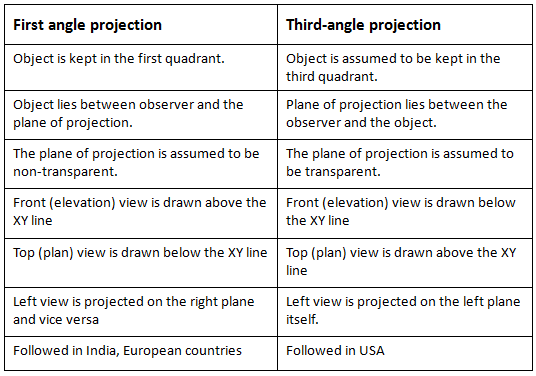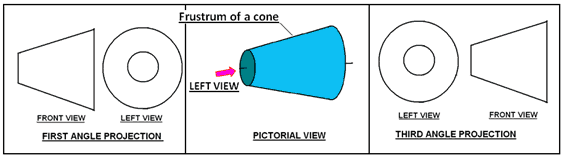### Projection Methods:

Projection Methods are used to show drawing on a paper . Projection Methods has mainly two types used  in Engineering Drawing :

1) First Angle Projection Method

2) Third Angle Projection Method

The principal projection planes and quadrants used to create drawings are shown in figure. The object can be considered to be in any of the four quadrant.Principal Projection Planes and Quadrants

First Angle Projection

In this the object in assumed to be positioned in the first quadrant and is shown in figure.Example of  First Angle Method

The object is assumed to be positioned in between the projection planes and the observer. The views are obtained by projecting the images on the respective planes. Note that the right hand side view is projected  on the plane placed at the left of the object. After projecting on to the respective planes, the bottom plane  and left plane is unfolded on to the front view plane.  i.e. the left plane is unfolded towards the left side to obtain the Right hand side view on the left side of the Front view and aligned with the Front view. The bottom plane is unfolded towards the bottom to obtain the Top view below the Front view and aligned with the Front View.

### Third Angle Projection

In the third angle projection method, the object is assumed to be in the third quadrant. i.e. the object behind vertical plane and below the horizontal plane. In this projection technique, Placing the object in the third quadrant puts the projection planes between the viewer and the object and is shown in figureExample of  Third Angle Method

### Difference between first- and third-angle projections

Either first angle projection or  third angle projection are used for engineering drawing.  Second angle projection and fourth angle projections are not used since the drawing becomes complicated.#### Symbol of projection

As per BIS standards, Drawing symbols for 1st angle and 3rd angle are shown in below figure. as previously explained that in case of first angle method the left hand side view is shown on right side of front view and vice verse.The symbol recommended by BIS is to draw the two sides of a frustum of a cone placed with its axis horizontal The left view is drawn.Symbol of first and third angle method